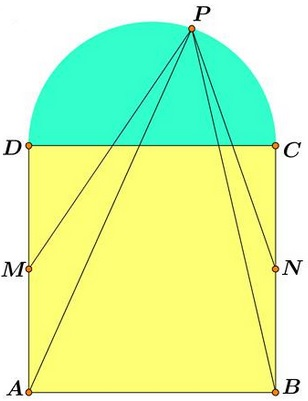# Square, Semicircle, and Other Curves Experimental Mathematics

### Clarification

The dashed curve is described by the equation $AP^{4}-BP^{4}=k(MP^{4}-NP^{4}),$ where parameter $m$ defines the position of $M$ on $AD;$ while $n$ defines the position of $N$ on $BC.$ When the box "Show trace" is checked the curve leaves a trace, to remove which press Ctrl-f, i.e., the two keys simultaneously. Nice pictures result, e.g,### Acknowledgment and History

This page grew from a previous one due to the comments and suggestions of Francisco Javier García Capitán (Córdoba, Spain) and Machó Bónis (Budapest, Hungary). The original problem has been posted by Miguel Ochoa Sanchez (Peru) and solved by Leo Giugiuc (Romania) who also suggested its first extension.

The original problem

$P$ is a point on the semicircle with diameter $CD$ constructed on a side of the square $ABCD.$ $M$ is the midpoint of $AD,$ $N$ the midpoint of $CD.$$\displaystyle\frac{AP^{4}-BP^{4}}{MP^{4}-NP^{4}}=2.$

Leo Giugiuc observed that the identity holds on the whole circle.

Francisco Javier García Capitán suggested replacing $2$ on the right of the equation with an arbitrary real $k$ and observed that the resulting equation (in $P)$ is that of a circle with radius $\displaystyle r=\frac{-k^{2}+3k-1}{2|r-1|}$ which is a real circle for $\phi -1\lt\sqrt{r}\lt\phi,$ where $\phi$ is the Golden Ratio.

Machó Bónis observed that the resulting equation is third degree (because the fourth powers of both $x$ and $y$ cancel out in both the numerator and the denominator of the fraction on the left) and that the straight vertical line through the center of the square is a part of the solution which, when factored out, leaves a second degree equation - a conic. He also suggested that GeoGebra has a facility to handle implicitly defined curves which led the above applet.